Search

About 148 Search Results Matching Types of Worksheet, Worksheet Section, Generator, Generator Section, Subjects matching Math, Grades matching 4th Grade, Similar to Valentine's Day Worksheet - Counting Eighteen, Page 2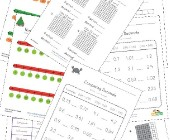Decimal Worksheets

This collection of free decimal worksheets will he...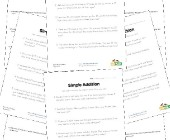Word Problem Worksheets

Help children develop their problem solving skills...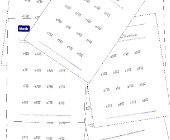Simple Division Worksheets

This group of worksheets makes a good introduction...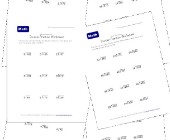Division Practice Worksheets

This collection of division practice worksheets is...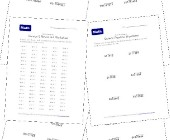Long Division Worksheets

Help kids practice long division with this set of ...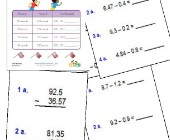Subtracting Decimals Worksheets

These worksheets will help children practice decim...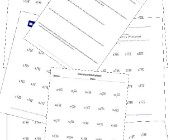Division Worksheets

Help kids learn division with this collection of m...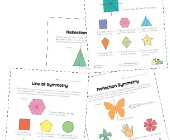Symmetry Worksheets

Help kids learn about the concept of symmetry with...